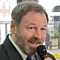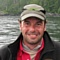# Project Management Central

We're used to using a simple sum function to add the EMV of multiple risks together. Would it be any more or less appropriate to use a SQRT(SUMSQ) function instead?Network:6Would the square root of the sum of the squares (evidently a common function in statistics) address the reduced likelihood of all of the identified risks hitting together better than simply summing the EMVs of all of those risks as we typically do?
Sort By:Network:150James,
I don't think it would be more appropriate. The root mean squares calculation gives you variance based on a number of measured data points. If you're applying it to a number of risks to estimate a variance, the calculation assumes each risk actually happened one time. They haven't occurred however, and they don't have equal probability.

What I have seen done instead is use some basic assumptions like 50% of the risks will become issues and we will achieve 30% of the opportunities (or whatever you think fits your situation). That takes into account both the magnitude, and the probability of the risks.Network:1306Keith -

I'm not sure I'd further pro-rate the EMV calculations as probability of occurrence is already built in unless you are dealing with a very pessimistic set of stakeholders who are expecting the worst when they are determining probability and impact values.

KironNetwork:150Kiron,
I think I see what he's trying to do. In addition to the PV which will have a variance from EV, there are other risks that could change the existing PV. How do you show the potential variance including those risks that aren't part of the PV?

If you add all the risks together, you are super pessimistic and assume all risks occur and you realize 100% of the impact. Some might occur but all probably won't so how do you reflect that in the total risk? RMS is used in statistical error analysis to come up with a total expected variance, knowing some estimates will be high while others are low offsetting each other somewhat.

I would agree that the total risk in a project is going to be lower than the "everything went wrong" scenario. I've seen other ways to try and estimate the total variance including both known work and risks but I don't see the RMS formula as a statistically valid way to show that.
KeithNetwork:12506You may need to do so, but generally, you do not need it. It is a little bit complicated and should be investigated based on situation, assumption and etc.